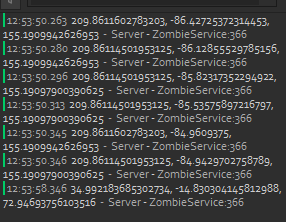# Tween is going nowhere near goal

i have a tween where it was working but then i moved it to another script to optimize it and now it is no longer working (WITH NO CHANGES)

video:

``````zombie.climbing = true
root.Anchored = true

-- time = distance / speed
local targetPos = Vector3.new(root.Position.X, target.Position.Y, root.Position.Z)
local dist = (root.Position - targetPos).Magnitude
local t = dist / humanoid.WalkSpeed
local info = TweenInfo.new(t, Enum.EasingStyle.Linear, Enum.EasingDirection.In)
local goal = { Value = CFrame.new(root.Position.X, root.Position.Y + dist, root.Position.Z) }

local p = Instance.new("Part", workspace)
p.CFrame = goal.Value
p.Anchored = true
p.CanQuery = false
p.CanCollide = false

local cf = Instance.new("CFrameValue")

local tween = TweenService:Create(cf, info, goal)
tween:Play()

local c = cf.Changed:Connect(function()
zombie.char:PivotTo(CFrame.new(cf.Value.Position))
end)

local connection
local ended = false
local function End()
if ended then return end
ended = true

c:Disconnect()
cf:Destroy()

tween:Cancel()
connection:Disconnect()
zombie.char:PivotTo(CFrame.new(root.Position - root.CFrame:VectorToObjectSpace(Vector3.new(0, 0, 3))))
root.Anchored = false
zombie.climbing = false
end)

tween:Destroy()
end

connection = tween.Completed:Connect(function()
End()
end)